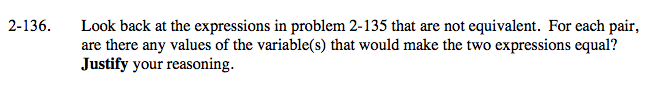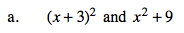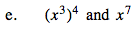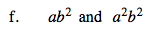### Home > A2C > Chapter Ch2 > Lesson 2.2.2 > Problem2-136

2-136.

Look back at the expressions in problem 2-135 that are not equivalent. For each pair, are there any values of the variable(s) that would make the two expressions equal? Justify your reasoning. 2-136 HW eTool (Desmos). Homework Help ✎Click the link at right for the full version of the eTool: A2C 2-136 HW eToolMultiply the first expression using a generic rectangle.

Set the expressions equal to each other and solve for x.

The equations are equal only if x = 0.Set the expressions equal to each other and simplify.

This is equal only if x = 0 or x = 1.See part (e).